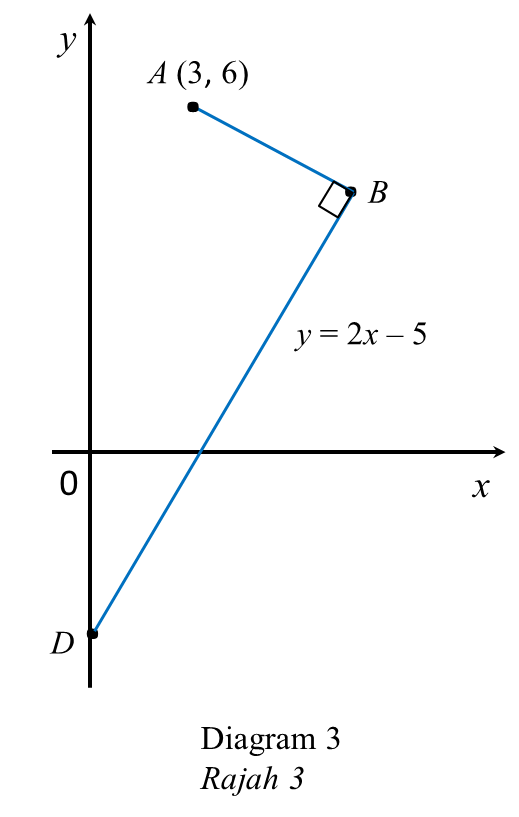\

# 7.8.5 Coordinate Geometry Long Question (Question 9)

Question 9:
Solution by scale drawing is not accepted.Diagram 3 shows three points, A, B and D in a Cartesian plane. The straight line AB is perpendicular to straight line BD which intersect the y-axis at D. The equation of the line BD is y = 2x – 5.
(a) Find the equation of the straight line AB. [2 Marks]
(b) The straight line AB is extended to a point C such that AB : BC= 2 : 3. Find the coordinates of C.    [3 Marks]
(c) Point P moves such that its distance from A is equal to its distance from B. Find the equation of the locus P.    [2 Marks]

Solution:
(a)
= 2x – 5
mBD = 2, mAB = –½
– 6 = –½ (x – 3)
2y – 12 = –x + 3
2y = –x + 15

(b)
= 2x – 5 ----- (1)
2y = –x + 15 ----- (2)
Substitute (1) into (2),
2 (2x – 5) = –x + 15
4x – 10 = –x + 15
5x = 25
x = 5
When x = 5, From (1)
= 2(5) – 5 = 5
B = (5, 5)AB : BC = 2 : 3
Let coordinates of point C = (h, k)

(c)
Let point = (x, y)
√(x – 3)2+ (y – 6)2 = √(x – 5)2 + (y – 5)2
(x – 3)2+ (y – 6)2 = (x – 5)2 + (y – 5)2  ← (Square for the both sides)
x2– 6x + 9 + y2 – 12y + 36 = x2 – 10x + 25 + y2 – 10y + 25
4x – 2y– 5 = 0